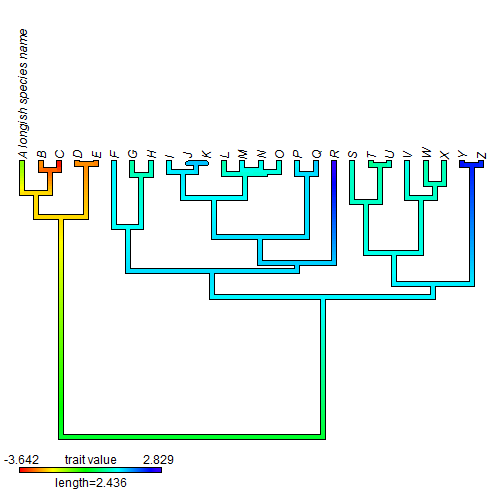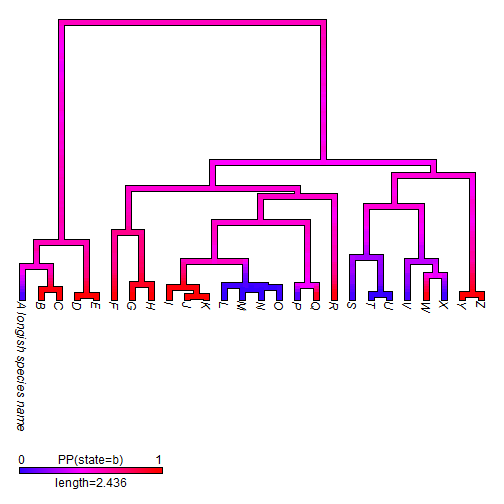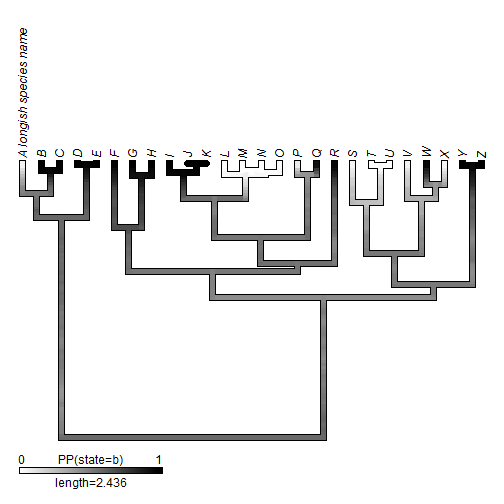## Monday, February 13, 2017

### Plotting up- and down-facing `"contMap"` & `"densityMap"` trees

Yesterday, I posted on plotting upward & downward facing trees with `plotSimmap` (and the wrapping function `plotTree`).

The reason I programmed this is to eventually extend this functionality to the methods that use `plotSimmap` internally - a long list that includes such functions as `plot.contMap` (& `contMap`) and `plot.densityMap` (& `densityMap`). These functions seem to be quite popular so I thought I'd do them first.

The biggest pain in the butt for these methods was not getting the phylogeny oriented correctly - after yesterday's phytools update, that part was easy. The hard part was getting the color-bar legend correctly positioned & sized. The reason this turned out to be challenging was because our plotting region is not square - meaning that x & y dimensions can have different scales. When our legend & branch lengths point in the same direction (right-to-left or left-to-right) we have nothing to worry about; however if we want to plot our tree upward or downward, but our legend (for the vertical branches) horizontally, then we start to have problems. To make this happen, we have to take advantage of `par("pin")` which gives us the relative dimensions of our plotting area in x & y - but in inches, not in our plotting units (these are given by `par("usr")`, by the way).

The updates can be seen on GitHub here.

Let's try a demo. Here I have simulated a simple tree, but added one longish tip label to make sure that the sizing of our plotting area to accomodate tip labels is working properly. (As mentioned in an earlier post, this is one of the other challenging thing about plotting phylogenies.)

``````library(phytools)
packageVersion("phytools")
``````
``````##  '0.5.76'
``````
``````tree
``````
``````##
## Phylogenetic tree with 26 tips and 25 internal nodes.
##
## Tip labels:
##  A longish species name, B, C, D, E, F, ...
##
## Rooted; includes branch lengths.
``````
``````x
``````
``````## A longish species name                      B                      C
##             -1.3444974             -2.7182556             -3.6415576
##                      D                      E                      F
##             -2.7367692             -2.9094996              1.0659075
##                      G                      H                      I
##              0.4134942              0.6560451              0.9139865
##                      J                      K                      L
##              1.3646047              1.3643494              0.4296533
##                      M                      N                      O
##              0.9882680              0.8843809              0.7174877
##                      P                      Q                      R
##              1.0307320              1.3304640              2.8291197
##                      S                      T                      U
##              0.6508031              0.1046013              0.7843597
##                      V                      W                      X
##              0.8382973              0.4644528              0.5338497
##                      Y                      Z
##              2.5244863              2.2260855
``````
``````obj<-contMap(tree,x,plot=FALSE)
obj
``````
``````## Object of class "contMap" containing:
##
## (1) A phylogenetic tree with 26 tips and 25 internal nodes.
##
## (2) A mapped continuous trait on the range (-3.641558, 2.82912).
``````
``````plot(obj,direction="upwards")
``````Next, using `densityMap`:

``````trees<-make.simmap(tree,y,nsim=100)
``````
``````## make.simmap is sampling character histories conditioned on the transition matrix
##
## Q =
##            a          b
## a -0.9922819  0.9922819
## b  0.9922819 -0.9922819
## (estimated using likelihood);
## and (mean) root node prior probabilities
## pi =
##   a   b
## 0.5 0.5
``````
``````## Done.
``````
``````obj<-densityMap(trees,plot=FALSE)
``````
``````## sorry - this might take a while; please be patient
``````
``````obj
``````
``````## Object of class "densityMap" containing:
##
## (1) A phylogenetic tree with 26 tips and 25 internal nodes.
##
## (2) The mapped posterior density of a discrete binary character with states (a, b).
``````
``````plot(obj,direction="downwards",lwd=5,outline=TRUE)
````````````obj<-setMap(obj,c("white","black"))
plot(obj,direction="upwards",lwd=5,outline=TRUE)
``````Neat.

The data for this exercise were simulated as follows:

``````tree<-pbtree(n=26,tip.label=LETTERS)
tree\$tip.label<-"A longish species name"
x<-fastBM(tree)
Q<-matrix(c(-1,1,1,-1),2,2)
rownames(Q)<-colnames(Q)<-letters[1:2]
y<-sim.history(tree,Q)\$states
``````